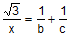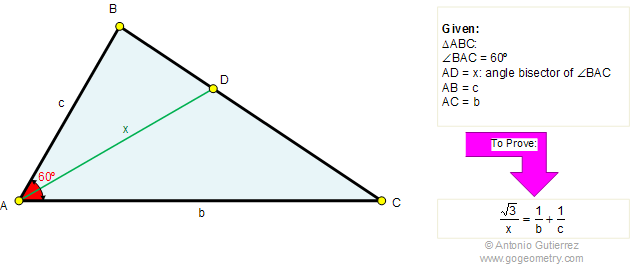Problem 302. Triangle, Angle bisector, 60 Degrees. Level: High School, SAT Prep, College geometry. In a triangle ABC, the angle BAC is equal to 60 degrees. If AB = c, AC = b, and AD = x. Prove that.Recent Additions